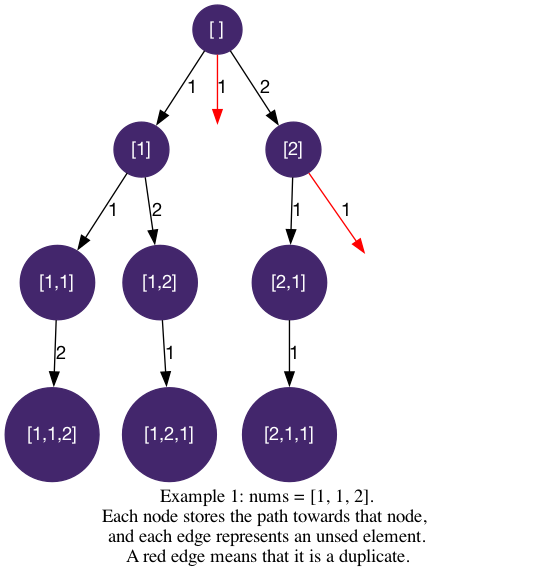# LeetCode Permutations II Solution

Given a collection of numbers, `nums`, that might contain duplicates, return all possible unique permutations in any order.

Example 1:

Input: `nums = [1,1,2]`

Output: `[[1,1,2], [1,2,1], [2,1,1]]`

Example 2:

Input: `nums = [1,2,3]`

Output: `[[1,2,3],[1,3,2],[2,1,3],[2,3,1],[3,1,2],[3,2,1]]`

Constraints:

• `1 <= nums.length <= 8`
• `-10 <= nums[i] <= 10`

## Solution

Let's fill in the logic for backtracking1:

• `path_length`: indicates the number of elements in `path`
• `used`: an array storing the status of whether an element is used.
• `is_leaf`: `path_length == len(nums)`, when `path_length` is the same as `nums`'s length.
• `get_edges` and `is_valid`: all elements that are unused (`used[i]==Flase`).

In the implementation, we use an array `used` to store the whether an element has been used or not. Everytime we use a number, we add it into the `path` and we mark it `used` (`used[idx] = True`). Since there are duplicate values, let's apply methods in Deduplication to deduplicate the results. we want to sort the `nums` array so that duplicate elements are consecutive, and only proceed when the current element is the first of its occurrance.

#### Implementation

``````1def permuteUnique(self, nums: List[int]) -> List[List[int]]:
2    used = [False] * len(nums)
3    ans = []
4    nums.sort()
5
6    def dfs(path_length, path):
7        if path_length == len(nums):
8            ans.append(path[:])
9            return
10
11        for i in range(0, len(nums)):
12            if used[i]: continue
13            if(i > 0 and nums[i] == nums[i-1] and not used[i-1]): continue
14            path.append(nums[i])
15            used[i] = True
16            dfs(path_length + 1, path)
17            used[i] = False
18            path.pop()
19
20    dfs(0, [])
21    return ans``````